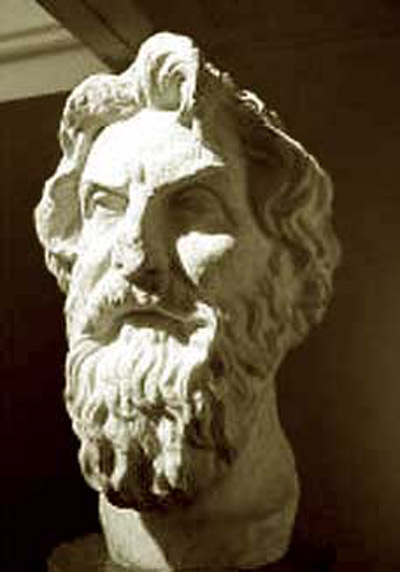A# Aristarchus of Samos (c. 310–c. 230 BC)Aristarchus measured the relative distances of the Sun and Moon. When the Moon is at its first quarter (A), the angle it makes with the Sun (C) is near 90°. By measuring the angle of the Earth (B) Aristarchus could determine from the triangle the relative distances from Earth. He found the angle to be 87° instead of the true value of 89° 52'; but a small error leads to a large discrepancy in the ratio of the distances of the Sun and Moon respectively from Earth. Aristarchus' ratio was 19:1; the true ratio is 390:1.Aristarchus of Samos was a Greek mathematician and astronomer, born on the island of Samos, who was the first to propose a heliocentric theory, with Earth revolving around the Sun. To explain the lack of observed stellar parallax, he argued that the stars must lie very far away. However, his new scheme for the Solar System found little support at a time when Aristotle’s geocentric model was in favor and the idea of a moving Earth was frowned upon.

Aristarchus applied modern geometric methods to measuring the size of celestial bodies. From a lunar eclipse, he concluded that the radius of the Moon is half that of Earth (actually it is 0.28 times as big). He measured the Moon’s angular diameter to be 2° (actually 0.5°) and calculated the Earth-Moon distance to be 114.6 Earth radii (actually 60.4). By noticing that the Sun and Moon have equal angular diameters during a solar eclipse, he estimated that the Sun’s distance was 19.1 times the Moon’s (actually 390 times). Aristarchus also argued that the lack of observed annual parallax in the fixed stars could be explained, within his heliocentric model, by assuming that the distance to the fixed stars is very much larger than the size of Earth's orbit. The same argument was made by Nicholas Copernicus, seventeen centuries later.

Aristarchus is believed to have studied and lived in Alexandria, where he died c. 250 BC. A mathematician at least as much as an astronomer, he belonged to the so-called Pythagorean school of thought, which sought to understand the universe in terms of geometrical and arithmetical relationships. His only surviving text is his Treatise on the Sizes and Distances of the Sun and Moon. However, largely through the writings of Archimedes and Plutarch, Aristarchus is known to have been the first proponent of the heliocentric hypothesis.